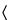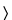Cupric Sulfate
(kue' prik sul' fate).

CuSO4·5H2O 249.69

CuSO4 159.61
Sulfuric acid, copper(2+) salt (1:1), pentahydrate;
Copper(2+) sulfate (1:1) pentahydrate[7758-99-8].
Anhydrous[7758-98-7].
DEFINITION
Cupric Sulfate, dried at 250to constant weight, contains NLT 98.5% and NMT 100.5 % of CuSO4.
IDENTIFICATION
A solution (1 in 10) meets the requirements.
A solution (1 in 10) meets the requirements.
ASSAY
•  Procedure
Sample solution:  Place 650 mg of Cupric Sulfate in a weighed container fitted with a ground-glass stopper. Dry, allow to cool in a desiccator, and weigh again to obtain the weight of the sample. Dissolve in 50 mL of water.
Analysis:  To the Sample solution add 4 mL of 6 N acetic acid and 3 g of potassium iodide. Titrate the liberated iodine with 0.1 N sodium thiosulfate VS, adding about 2 g of potassium thiocyanate and 3 mL of starch TS as the endpoint is approached. Perform a blank determination, and make any necessary correction. Each mL of 0.1 N sodium thiosulfate is equivalent to 15.96 mg of cupric sulfate (CuSO4).
Acceptance criteria:  98.5%–100.5% on the dried basis
IMPURITIES
•  Limit of Sodium
Sample stock solution:  Transfer 40.0 g of Cupric Sulfate to a 200-mL volumetric flask, add water, and swirl to dissolve. Add 5 mL of nitric acid, and dilute with water to volume. This solution contains 0.2 g/mL of cupric sulfate.
Sample solutions:  To three 25-mL volumetric flasks add a volume of Sample stock solution equivalent to the Sample Weight given in Table 1. To two of the flasks add the amounts of reference analyte ion specified in Table 1. Add 2 mL of potassium chloride solution (1 in 20) to each flask, and dilute with water to volume.
Analysis:  Using atomic absorption spectrophotometry (see Spectrophotometry and Light-Scattering851), analyze the Sample solutions by the method of standard addition analysis given in Table 1.
Acceptance criteria:  NMT 0.02%
Table 1
Limit Test Wavelength
(nm)
Sample
Weight
(g)
Reference
Ion
(mg)
Flame Type Background
Correction
Sodium 589.0 0.05 0.005/0.01 Air–
acetylene
No
Potassium 766.5 0.4 0.02/0.04 Air–
acetylene
No
Calcium 422.7 0.8 0.02/0.04 Nitrous oxide–
acetylene
No
Iron 248.3 4.0 0.12/0.24 Air–
acetylene
Yes
Nickel 232.0 4.0 0.10/0.20 Air–
acetylene
No
•  Limit of Potassium
Sample stock solution:  Prepare as directed in the test for Limit of Sodium.
Sample solutions:  To three 25-mL volumetric flasks add a volume of Sample stock solution equivalent to the Sample Weight given in Table 1. To two of the flasks add the amounts of reference analyte ion specified in Table 1, and dilute with water to volume.
Analysis:  Using atomic absorption spectrophotometry (see Spectrophotometry and Light-Scattering851), analyze the Sample solutions by the method of standard addition analysis given in Table 1.
Acceptance criteria:  NMT 0.01%
•  Limit of Calcium
Sample stock solution:  Prepare as directed in the test for Limit of Sodium.
Sample solutions:  To three 25-mL volumetric flasks add a volume of Sample stock solution equivalent to the Sample Weight given in Table 1. To two of the flasks add the amounts of reference analyte ion specified in Table 1, and dilute with water to volume.
Analysis:  Using atomic absorption spectrophotometry (see Spectrophotometry and Light-Scattering851), analyze the Sample solutions by the method of standard addition analysis given in Table 1.
Acceptance criteria:  NMT 0.005%
•  Limit of Iron
Sample stock solution:  Prepare as directed in the test for Limit of Sodium.
Sample solutions:  To three 25-mL volumetric flasks add a volume of Sample stock solution equivalent to the Sample Weight given in Table 1. To two of the flasks add the amounts of reference analyte ion specified in Table 1, and dilute with water to volume.
Analysis:  Using atomic absorption spectrophotometry (see Spectrophotometry and Light-Scattering851), analyze the Sample solutions by the method of standard addition analysis given in Table 1.
Acceptance criteria:  NMT 0.003%
•  Limit of Nickel
Sample stock solution:  Prepare as directed in the test for Limit of Sodium.
Sample solutions:  To three 25-mL volumetric flasks add a volume of Sample stock solution equivalent to the Sample Weight given in Table 1. To two of the flasks add the amounts of reference analyte ion specified in Table 1, and dilute with water to volume.
Analysis:  Using atomic absorption spectrophotometry (see Spectrophotometry and Light-Scattering851), analyze the Sample solutions by the method of standard addition analysis given in Table 1.
Acceptance criteria:  NMT 0.005%
SPECIFIC TESTS
Dry a sample at 250to constant weight: it loses 33.0%–36.5% of its weight.